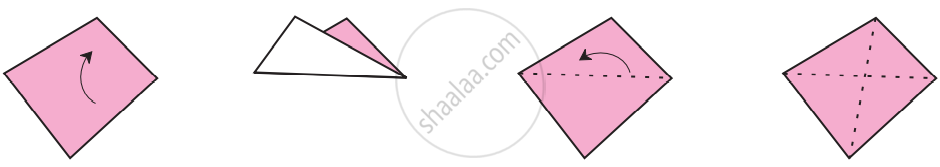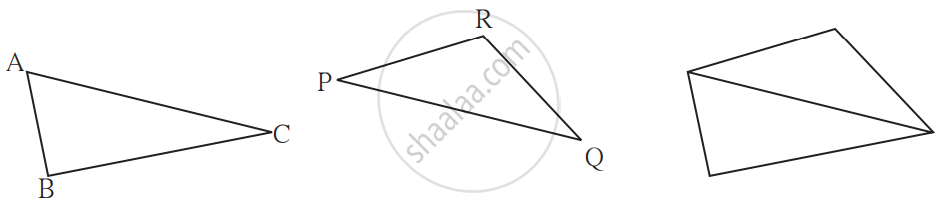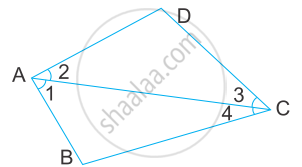# Sum of Four Angles of a Quadrilateral:

• Cut out a paper in the shape of a quadrilateral.
• Make folds in it that join the vertices of opposite angles.• Take two triangular pieces of paper such that one side of one triangle is equal to one side of the other.
• Let us suppose that in ∆ABC and ∆PQR, sides AC and PQ are the equal sides.• Join the triangles so that their equal sides lie side by side.
• We used two triangles to obtain a quadrilateral. The sum of the three angles of a triangle is 180°.
• Hence, The sum of the measures of the four angles of a quadrilateral is 360°.

# Angle Sum Property of a Quadrilateral:

Theorem: The sum of the angles of a quadrilateral is 360°.Construction: This can be verified by drawing a diagonal AC and dividing the quadrilateral into two triangles.

Proof:

Let ABCD be a quadrilateral and AC be diagonal.

In △ ABC,

You know that,
∠ B + ∠ BAC + ∠ BCA = 180°........(1)
∠ D + ∠ DAC + ∠ DCA = 180°........(2)
Adding (1) and (2), we get,
∠ B + ∠ BAC + ∠ BCA + ∠ D + ∠ DAC + ∠ DCA = 180° + 180°

Also, ∠ BAC + ∠ DAC = ∠ A and ∠ BCA + ∠ DCA = ∠ C

So, ∠ A + ∠ B + ∠ C + ∠ D = 180° + 180°= 360°

i.e., The sum of the angles of a quadrilateral is 360°.

If you would like to contribute notes or other learning material, please submit them using the button below.

### Shaalaa.com

Prove Sum of Interior Angles of a Quadrilateral are 360 Degrees [00:03:05]
S
0%